Function Repository Resource:

# CircularWinder

Create a rotatable arrow handle

Contributed by: Wolfram Staff
 ResourceFunction["CircularWinder"][θ] represents a circular winder graphics object with an arrow rotated by the angle θ. ResourceFunction["CircularWinder"][Dynamic[θ]] takes the angle position to be the dynamically updated current value of θ, with this value being reset if the winder arrow is moved.

## Details

ResourceFunction["CircularWinder"] is a wrapper function for Experimental`AngularSlider.
The spiral in the center of the circular winder corresponds to the absolute value of the rotation angle.
ResourceFunction["CircularWinder"][Dynamic[]] is also accepted, with arguments suitable for Dynamic.

## Examples

### Basic Examples (2)

Set up a circular winder with a movable arrow:

 In:=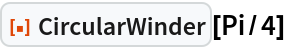Out=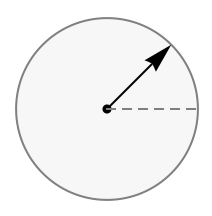Set up a circular winder that defines the value of the angle:

 In:=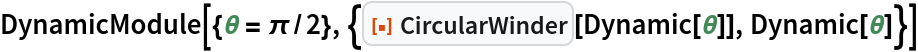Out=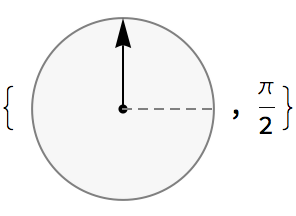### Scope (2)

A continuous circular winder:

 In:=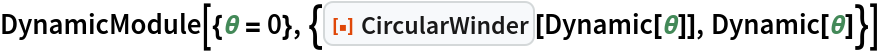Out=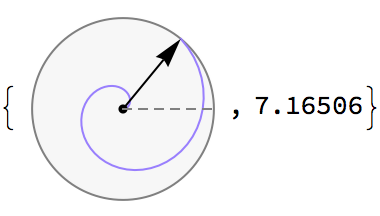A discrete circular winder:

 In:=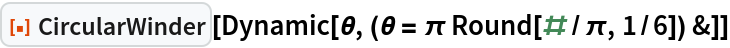Out=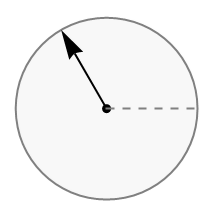Set up a discrete circular winder that defines the value of the angle:

 In:=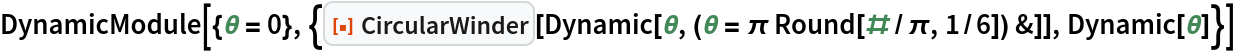Out=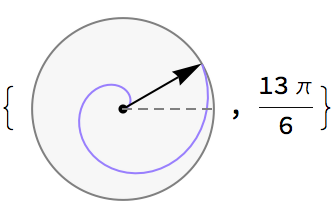## Version History

• 2.0.0 – 17 May 2021
• 1.0.0 – 04 November 2019